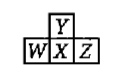A compound contains three elements A, B and C, if the oxidation number of A =+2, B =+5 and C =-2, the possible formula of the compound is :

(a) ${A}_{3}{\left({B}_{4}C\right)}_{2}$                                  (b) ${A}_{3}{\left(B{C}_{4}\right)}_{2}$

(c) ${A}_{2}{\left(B{C}_{3}\right)}_{2}$                                  (d) $AB{C}_{2}$

Concept Questions :-

Nature of Compound
High Yielding Test Series + Question Bank - NEET 2020

Difficulty Level:

Consider the following four elements, which are represented according to long form of periodic table.Here W, Y and Z are left, up and right elements with respect to the element 'X' and 'X' belongs to 16th group and 3rd period.Then according to given information the incorrect statement regarding given elements is:

(a) Maximum electronegativity :Y              (b) Maximum catenation property :X

(c) Maximum electron affinity :Z               (d) Y exhibits variable covalency

Concept Questions :-

Electron Affinity (EA)
High Yielding Test Series + Question Bank - NEET 2020

Difficulty Level:

The ground state electronic configurations of the elements, U,V,W,X,and Y (these symbols do not have any chemical significance) are as follows:

Determine which sequence of elements satisfy the following statements :

(i) Element forms a carbonate which is not decomposed by heating

(ii) Element is most likely to form coloured ionic compounds

(iii) Element has largest atomic radius

(iv) Element forms only acidic oxide

(a) V W Y U                                   (b) V X Y W

(b) V W Y X                                   (d) V X W U

Concept Questions :-

Electronic Configuration
High Yielding Test Series + Question Bank - NEET 2020

Difficulty Level:

The electronic configuration of four elements are :which one will show variable oxidation state?

(I) $\left[Kr\right]5{s}^{1}$                                 (II) $\left[Rn\right]5{f}^{14}6{d}^{1}7{s}^{2}$
(III)$\left[Ar\right]3{d}^{10}4{s}^{2}4{p}^{5}$                    (IV) $\left[Ar\right]3{d}^{6}4{s}^{2}$

Concept Questions :-

Electronic Configuration
High Yielding Test Series + Question Bank - NEET 2020

Difficulty Level:

If period number and group number of any representative element(s) are same then which of the following statement is incorrect regarding such type element(s) in their ground state? (Period number and group number are according to modern form of periodic table)

(a) The possible value of principal quantum number is 2

(b) The possible value of azimuthal quantum number is zero

(c) The possible value of magnetic quantum number is 1

(d) The species could be paramagnetic

High Yielding Test Series + Question Bank - NEET 2020

Difficulty Level:

How does the energy gap between successive energy levels in an atom vary from low to high n values?

(a) All energy gaps are the same

(b) The energy gap decreases as n increases

(c) The energy gap increases as n increases

(d) The enenrgy gap changes unpredictably as n increases

Concept Questions :-

Ionization Energy (IE)
High Yielding Test Series + Question Bank - NEET 2020

Difficulty Level:

Which of the following properties of the alkaline earth metals increase from Be to Ba?

(i) Atomic radius        (ii) Ionisation energy        (iii) Nuclear charge

(a) (i) and (ii)                                 (b) (i) and (iii)

(c) (ii) and (iii)                                (d) (i), (ii) and (iii)

Concept Questions :-

Atomic Size
High Yielding Test Series + Question Bank - NEET 2020

Difficulty Level:

Incorrect order of ionic size is :

(a)$L{a}^{3+}>G{d}^{3+}>E{u}^{3+}>L{u}^{3+}$           (b)${V}^{2+}>{V}^{3+}>{V}^{4+}>{V}^{5+}$

(c)$T{l}^{+}>I{n}^{+}>S{n}^{2+}>S{b}^{3+}$                (d)${K}^{+}>S{c}^{3+}>{V}^{5+}>M{n}^{7+}$

Concept Questions :-

Atomic Size
High Yielding Test Series + Question Bank - NEET 2020

Difficulty Level:

Consider the following changes:

The second ionization energy of M could be calculated from the energy values associated with :

(a) 1+3+4                  (b) 2-1+3

(c) 1+5                      (d) 5-3

Concept Questions :-

Ionization Energy (IE)
High Yielding Test Series + Question Bank - NEET 2020

Difficulty Level:

Which of the following statements is/are wrong?

(a) van der Waals' radius of iodine is more than its covalent radius

(b) All isoelectronic ions belong to same period of the periodic table

(c) $I.E{.}_{1}$ of N is higher than that of O while $I.E{.}_{2}$ of O is higher than that of N

(d) The electron affinity N is almost zero while that of P is 74.3 kJ $mo{l}^{-1}$

Concept Questions :-

History